## ML Aggarwal Class 6 Solutions for ICSE Maths Chapter 4 Playing with Numbers Objective Type Questions

Mental Maths
Question 1.
Fill in the blanks:
(i) The only natural number which has exactly one factor is ……….
(ii) The only prime number which is even is ……….
(iii) The HCF of two co-prime numbers is ……….
(iv) Two perfect numbers are ………. and ……….
(v) The only prime-triplet is ……….
Solution:
(i) The only natural number which has exactly one factor is 1.
(ii) The only prime number which is even is 2.
(iii) The HCF of two co-prime numbers is 1.
(iv) Two perfect numbers are 6 and 28.
(v) The only prime-triplet is 3, 5, 7.

Question 2.
State whether the following statements are true (T) or false (F):
(i) Every natural number has a finite number of factors.
(ii) Every natural number has an infinite number of its multiples.
(iii) There are infinitely many prime numbers.
(iv) If two numbers are separately divisible by a number, then their difference is also divisible by that number.
(v) LCM of two prime numbers equals their product.
(vi) LCM of two co-prime numbers equals their product.
Solution:
(i) Every natural number has a finite number of factors. True
(ii) Every natural number has an infinite number of its multiples. True
(iii) There are infinitely many prime numbers. True
(iv) If two numbers are separately divisible by a number, then their difference is also divisible by that number. True
(v) LCM of two prime numbers equals their product. True
(vi) LCM of two co-prime numbers equals their product. True

Question 3.
State whether the following statements are true or false. If a statement is false, justify your answer.
(i) The sum of two prime numbers is always an even number.
(ii) The sum of two prime numbers is always a prime number.
(iii) The sum of two prime numbers can never be a prime number
(iv) No odd number can be written as the sum of two prime numbers.
(v) If two numbers are co-prime, then atleast one of them must be prime.
(vi) If a number is divisible by 18, it must be divisible by 3 and 6 both.
(vii) If a number is divisible by 2 and 4 both, it must be divisible by 8.
(viii) If a number is divisible by 3 and 6 both, it must be divisible by 18.
(ix) HCF of an even number and an odd number is always 1.
Solution:
(i) The sum of two prime numbers is always an even number. False
Correct:
2 and 7 both are prime numbers but their sum = 2 + 7 = 9, which is an odd number.

(ii) The sum of two prime numbers is always a prime number. False
Correct:
3 and 5 both are prime numbers but their sum = 3 + 5 = 8, which is a composite number.

(iii) The sum of two prime numbers can never
be a prime number. False
Correct:
2 and 5 both are prime numbers but their sum = 2 + 5 = 7, which is a prime number.

(iv) No odd number can be written as the sum of two prime numbers. False
Correct:
13 is an odd number and 13 = 2+11, which is the sum of two prime numbers.

(v) If two numbers are co-prime, then atleast one of them must be prime. False
Correct :
8 and 15 are co-prime numbers but neither 8 is prime nor 15 is prime.

(vi) If a number is divisible by 18, it must be divisible by 3 and 6 both. True

(vii) If a number is divisible by 2 and 4 both, it
must be divisible by 8. False
Correct :
20 is divisible by 2 and 4 both but 20 is not divisible by 8.

(viii)If a number is divisible by 3 and 6 both, it must be divisible by 18. False
Correct:
12 is divisible by 3 and 6 both bu 12 is not divisible by 18.

(ix) HCF of an even number and an odd number is always 1. False
Correct:
6 is even and 9 is odd but HCF of 6 and 9 is 3.

Multiple Choice Questions
Choose the correct answer from the given four options (4 to 28):
Question 4.
All factors of 6 are
(a) 1, 6
(b) 2, 3
(c) 1, 2, 3
(d) 1, 2, 3, 6
Solution:
The factors of 6 are 1, 2, 3, 6 (d)

Question 5.
Which of the following is an odd composite number?
(a) 7
(b) 9
(c) 11
(d) 12
Solution:
9, is an odd composite number. (c)

Question 6.
The number of even numbers between 68 and 90 is
(a) 10
(b) 11
(c) 12
(d) 31
Solution:
The even numbers between 68 and 90 is 70, 72, 74, 76, 78, 80, 82, 84, 86, 88 = 10 numbers (a)

Question 7.
Which of the following is a prime number?
(a) 69
(b) 87
(c) 91
(d) 97
Solution:
Since, the factors of 97 are 1 and 97
97 is a prime number. (d)

Question 8.
Which of the following is a pair of twin- prime number?
(a) 19, 21
(b) 43, 47
(c) 59, 61
(d) 73, 79
Solution:
59, 61
Pairs of prime numbers whose difference is 2 are called twin-prime numbers. (c)

Question 9.
The number of distinct prime factors of the largest 4-digit number is
(a) 2
(b) 3
(c) 5
(d) none of these
Solution:
Largest 4 digit number = 99993 is prime factor. (b)

Question 10.
The number of distinct prime factors of the smallest 5-digit number is
(a) 2
(b) 4
(c) 6
(d) 8
Solution:
Smallest 5-digit number = 10000Number of distinct prime factors of smallest 5-digit number = 2 (a)

Question 11.
The sum of the prime factors of 1729 is
(a) 13
(b) 19
(c) 32
(d) 39
Solution: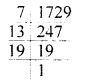Prime factors of 1729 are 7, 13 and 19 Sum of prime factors = 7 + 13 + 19 = 39 (d)

Question 12.
Which of the following is a pair of co-prime numbers?
(a) 8, 45
(b) 3, 18
(c) 5, 35
(d) 6, 39
Solution:
8, 15
The factors of 8 are 1, 2, 4, 8 The factors of 15 are 1, 3, 5, 15 The common factors of 8 and 15 is 1 They are co-prime. (a)

Question 13.
Every natural number has an infinite number of
(a) prime factors
(b) factors
(c) multiples
(d) none of these
Solution:
Multiples
e.g. Multiples of 2 = 2, 4, 6, 8, 10, 12, 14, 16, …….. (c)

Question 14.
Which of the following numbers is divisible by 4?
(a) 308594
(b) 506784
(c) 732106
(d) 9301538
Solution:
506784
Because the number formed by tens and ones digits is divisible by 4 i.e. 84 ÷ 4 = 21 (b)

Question 15.
Which of the following numbers is divisible by 8?
(a) 503786
(b) 505268
(c) 305678
(d) 703568
Solution:
703568
Because the number formed by hundred, tens
and ones digit is divisible by 8
i. e. 568 – 8 = 71 (d)

Question 16.
Which of the following numbers is divisible by 3?
(a) 50762
(b) 42063
(c) 52871
(d) 37036
Solution:
42063
Because sum of its digits is = 4 + 2 + 0 + 6 + 3 = 15 Which is divisible by 3 (b)

Question 17.
Which of the following numbers is divisible by 9?
(a) 972063
(b) 730542
(c) 785423
(d) 5612844
Solution:
972063
Because sum of digits
= 9 + 7 + 2 + 0 + 6 + 3 = 27 Which is divisible by 9 (a)

Question 18.
Which of the following numbers is divisible by 6?
(a) 560324
(b) 650374
(c) 798653
(d) 750972
Solution:
750972
Because sum of its digit
= 7 + 5 + 0 + 9 + 7 + 2 = 30 Which is divisible by 3.
Hence it is divisible by 6. (d)

Question 19.
The digit by which ‘*’ should be replaced in 54 * 281 so that the number formed is divisible by 9 is
(a) 6
(b) 7
(c) 8
(d) 9
Solution:
For a number to be divisible by 9, sum of its digits should be divisible by 9.
Sum of given digits in 54 * 281
= 5 + 4 + 2 + 8 + 1 = 20.
If we add 7, it becomes 27, which is divisible by 9.
∴ * is to be replaced by 7. (b)

Question 20.
The digit by which should be replaced in 7254 * 98 so that the number formed is divisible by 22 is
(a) 0
(b) 1
(c) 2
(d) 6
Solution:
For a number to be divisible by 22, sum of its digits should be divisible by 2 and by 11.
Since, the last digit of 7254 * 98 is 8, which is divisible by 2.
Now,
Sum of the digits at odd places = 7 + 5 + 8 = 20
Sum of the digits at even places = 9 + 4 + 2 = 15
∴ Their Difference = 20 – 15 = 5
Since, 5 is not divisble by 11, so to make a number divisible by 11 we must add 6.
∴ * is to be replaced by 6 (d)

Question 21.
If a number is divisible by 5 and 6 both, then it may not be divisible by
(a) 10
(b) 15
(c) 30
(d) 60
Solution:
60 (d)

Question 22.
The number of common prime factors of 60, 75 and 105 is
(a) 2
(b) 3
(c) 4
(d) 5
Solution:
60, 75 and 105=2 × 2 × 3 × 5 × 5 × 7 = 2 (a)

Question 23.
The H.C.F. of 144 and 198 is
(a) 6
(b) 9
(c) 12
(d) 18
Solution:
H.C.F. of 144 = 2 × 2 × 2 × 2 × 3 × 3
H.C.F. of 198 = 2 × 3 × 3 × 11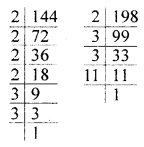∴ H. C. F of 144 and 198
= 2 × 3 × 3 = 18 (d)

Question 24.
The L.C.M. of 30 and 45 is
(a) 15
(b) 30
(c) 45
(d) 90
Solution:
L.C.M. of 30 and 45 is 90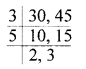∴ L.C.M. = 3 × 5 × 2 × 3 = 90 (d)

Question 25.
The L.C.M. of 4 and 44 is
(a) 4
(b) 11
(c) 44
(d) 176
Solution:
LCM of 4 and 44 is 44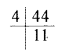∴ L.C.M = 4 × 11 =44 (c)

Question 26.
The LCM of 7 and 13 is 1
(a) 1
(b) 7
(c) 13
(d) 91
Solution:
LCM of 7 and 13 is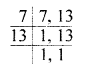LCM of 7 and 13 is 91
L.C.M. = 7 × 13 = 91 (d)

Question 27.
If H.C.F. of two numbers is 15 and their product is 1575, then their L.C.M. is
(a) 15
(b) 105
(c) 525
(d) 1575
Solution:
Product of numbers =1575
H.C.F. = 15
We know,
L.C. M = $$\frac{\text { Product of numbers }}{\text { H.C.F. }}$$
$$=\frac{1575}{15}=105$$ (b)

Question 28.
If the LCM of two natural numbers is 180, then which of the following is not the HCF of the numbers?
(a) 45
(b) 60
(c) 75
(d) 90
Solution:
L.C.M. of 2 natural numbers = 180
We know that,
L.C.M. of 2 numbers is always exactly divisible by their H.C.F.
∴ Taking (a) 45 as H.C.F.Here remainder = 0
∴ 45 is H.C.F.
Now, taking (b) 60 as H.C.F.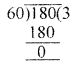Here remainder = 0
∴ 60 is also H.C.F.
Now, taking 75 as H.C.F.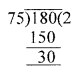Here, remainder = 30
i. e. remainder ≠ 0
Hence, 75 is not the H.C.F. of two natural numbers whose L.C.M. is 180

Value Based Questions
Question 1.
To teach the value of gratitude and appreciation to the students, a school organised a ‘Card Making’ activity in which the students were asked to make “THANK YOU CARDS” for the people who helped them in some way. Assorted cards were made with different titles. Their numbers are given below:
T cards for teachers = 120
F cards for friends = 540
S cards for servants = 90
P cards for parents = 240 and
G cards for grandparents = 150
(i) Find the HCF and LCM of all the different number of cards.
(ii) Find HCF and LCM of maximum and minimum number of cards.
(iii) Is the number of T-cards is a factor of number of P-cards?
Solution:
(i) HCF of 120, 540, 90, 240, 150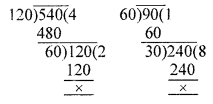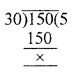∴ HCF = 30
Now, LCM of 120, 540, 90, 240 and 150 is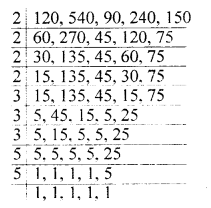∴ LCM = 2 × 2 × 2 × 2 × 3 × 3 × 3 × 5 × 5
= 10800

(ii) Maximum number of cards = 540
Minimum number of cards = 90
∴ HCF is as follow :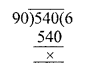Hence, HCF of 90 and 540 is 90
LCM of 90 and 540 is as follow :LCM = 2 × 2 × 3 × 3 × 3 × 5 = 540

(iii) Yes.
T cards = 120
P cards = 240
240 = 120 × 2

Higher Order Thinking Skills (Hots)
Question 1.
Write 2-digit odd numbers whose sum of digits is 8.
Solution:
17, 71, 35, 53

Question 2.
Write all pairs of 2-digit twin primes such that on changing the places of their digits, they still remain prime numbers.
Solution:
11, 13, 71, 73

Question 3.
There are just four natural numbers less than 100, which have exactly three factors. One of them is 25, what are the other three? What can be said about these numbers?
Solution:
Four natural numbers less than 100 which have three factors :
One of them is 25 = 1, 5, 25
Second is 49 = 1, 7, 49
Third is 9 = 1, 3, 9
Fourth is 4 = 1, 2, 4